# Classical mechanics

Classical mechanics
History · Timeline

Classical mechanics is used for describing the motion of macroscopic objects, from projectiles to parts of machinery, as well as astronomical objects, such as spacecraft, planets, stars, and galaxies. It produces very accurate results within these domains, and is one of the oldest and largest subjects in science, engineering and technology.

Besides this, many related specialties exist, dealing with gases, liquids, and solids, and so on. Classical mechanics is enhanced by special relativity for objects moving with high velocity, approaching the speed of light; general relativity is employed to handle gravitation at a deeper level; and quantum mechanics handles the wave-particle duality of atoms and molecules.

In physics, classical mechanics is one of the two major sub-fields of study in the science of mechanics, which is concerned with the set of physical laws governing and mathematically describing the motions of bodies and aggregates of bodies. The other sub-field is quantum mechanics.

## Terminology

The term classical mechanics was coined in the early twentieth century to describe the system of mathematical physics begun by Isaac Newton and many contemporary seventeenth-century workers, building upon the earlier astronomical theories of Johannes Kepler. These theories in turn were based on the precise observations of Tycho Brahe and the studies of terrestrial projectile motion of Galileo. In that sense, classical mechanics was divided from quantum physics and relativity, and some sources exclude so-called "relativistic physics" from that category. However, a number of modern sources do include Einstein's mechanics, which in their view represents classical mechanics in its most developed and most accurate form.

The initial stage in the development of classical mechanics is often referred to as Newtonian mechanics, and is associated with the physical concepts employed by and the mathematical methods invented by Newton himself, in parallel with Leibniz, and others. This is further described in the following sections. More abstract and general methods include Lagrangian mechanics and Hamiltonian mechanics. Much of the content of classical mechanics was created in the eighteenth and nineteenth centuries and extends considerably beyond (particularly in its use of analytical mathematics) the work of Newton.

## History

Some Greek philosophers of antiquity, among them Aristotle, may have been the first to maintain the idea that "everything happens for a reason" and that theoretical principles can assist in the understanding of nature. While, to a modern reader, many of these preserved ideas come forth as eminently reasonable, there is a conspicuous lack of both mathematical theory and controlled experiment, as we know it. These both turned out to be decisive factors in forming modern science, and they started out with classical mechanics.

An early experimental scientific method was introduced into mechanics in the 11th century by al-Biruni, who along with al-Khazini in the 12th century, unified statics and dynamics into the science of mechanics, and combined the fields of hydrostatics with dynamics to create the field of hydrodynamics. Concepts related to Newton's laws of motion were also enunciated by several other Muslim physicists during the Middle Ages. Early versions of the law of inertia, known as Newton's first law of motion, and the concept relating to momentum, part of Newton's second law of motion, were described by Ibn al-Haytham (Alhacen) and Avicenna. The proportionality between force and acceleration, an important principle in classical mechanics, was first stated by Hibat Allah Abu'l-Barakat al-Baghdaadi, and theories on gravity were developed by Ja'far Muhammad ibn Mūsā ibn Shākir, Ibn al-Haytham, and al-Khazini. It is known that Galileo Galilei's mathematical treatment of acceleration and his concept of impetus grew out of earlier medieval analyses of motion, especially those of Avicenna, Ibn Bajjah, and Jean Buridan.

The first published causal explanation of the motions of planets was Johannes Kepler's Astronomia nova published in 1609. He concluded, based on Tycho Brahe's observations of the orbit of Mars, that the orbits were ellipses. This break with ancient thought was happening around the same time that Galilei was proposing abstract mathematical laws for the motion of objects. He may (or may not) have performed the famous experiment of dropping two cannon balls of different masses from the tower of Pisa, showing that they both hit the ground at the same time. The reality of this experiment is disputed, but, more importantly, he did carry out quantitative experiments by rolling balls on an inclined plane. His theory of accelerated motion derived from the results of such experiments, and forms a cornerstone of classical mechanics.

As foundation for his principles of natural philosophy, Newton proposed three laws of motion, the law of inertia, his second law of acceleration, mentioned above, and the law of action and reaction, and hence laying the foundations for classical mechanics. Both Newton’s second and third laws were given proper scientific and mathematical treatment in Newton's Philosophiæ Naturalis Principia Mathematica, which distinguishes them from earlier attempts at explaining similar phenomena, which were either incomplete, incorrect, or given little accurate mathematical expression. Newton also enunciated the principles of conservation of momentum and angular momentum. In Mechanics, Newton was also the first to provide the first correct scientific and mathematical formulation of gravity in Newton's law of universal gravitation. The combination of Newton's laws of motion and gravitation provide the fullest and most accurate description of classical mechanics. He demonstrated that these laws apply to everyday objects as well as to celestial objects. In particular, he obtained a theoretical explanation of Kepler's laws of motion of the planets.

Newton previously invented the calculus, of mathematics, and used it to perform the mathematical calculations. For acceptability, his book, the Principia, was formulated entirely in terms of the long established geometric methods, which were soon to be eclipsed by his calculus. However it was Leibniz who developed the notation of the derivative and integral preferred today.

Newton, and most of his contemporaries, with the notable exception of Christiaan Huygens, worked on the assumption that classical mechanics would be able to explain all phenomena, including light, in the form of geometric optics. Even when discovering the so-called Newton's rings (a wave interference phenomenon), his explanation remained with his own corpuscular theory of light.

After Newton, classical mechanics became a principal field of study in mathematics as well as physics.

Some difficulties were discovered in the late nineteenth century that could be resolved only by more modern physics. Some of these difficulties related to compatibility with electromagnetic theory, and the famous Michelson-Morley experiment. The resolution of these problems led to the special theory of relativity, often included in the term classical mechanics.

A second set of difficulties related to thermodynamics. When combined with thermodynamics, classical mechanics leads to the Gibbs paradox of classical statistical mechanics, in which entropy is not a well-defined quantity. Black-body radiation was not explained without the introduction of quanta. As experiments reached the atomic level, classical mechanics failed to explain, even approximately, such basic things as the energy levels and sizes of atoms and the photo-electric effect. The effort at resolving these problems led to the development of quantum mechanics.

Since the end of the twentieth century, the place of classical mechanics in physics has been no longer that of an independent theory. Emphasis has shifted to understanding the fundamental forces of nature as in the Standard model and its more modern extensions into a unified theory of everything. Classical mechanics is a theory for the study of the motion of non-quantum mechanical, low-energy particles in weak gravitational fields.

## Description of the theory

The following introduces the basic concepts of classical mechanics. For simplicity, it often models real-world objects as point particles, objects with negligible size. The motion of a point particle is characterized by a small number of parameters: its position, mass, and the forces applied to it. Each of these parameters is discussed in turn.

In reality, the kind of objects which classical mechanics can describe always have a non-zero size. (The physics of very small particles, such as the electron, is more accurately described by quantum mechanics). Objects with non-zero size have more complicated behavior than hypothetical point particles, because of the additional degrees of freedom—for example, a baseball can spin while it is moving. However, the results for point particles can be used to study such objects by treating them as composite objects, made up of a large number of interacting point particles. The center of mass of a composite object behaves like a point particle.

### Displacement and its derivatives

 The SI derived units with kg, m and s displacement m speed m s−1 acceleration m s−2 jerk m s−3 specific energy m² s−2 absorbed dose rate m² s−3 moment of inertia kg m² momentum kg m s−1 angular momentum kg m² s−1 force kg m s−2 torque kg m² s−2 energy kg m² s−2 power kg m² s−3 pressure kg m−1 s−2 surface tension kg s−2 irradiance kg s−3 kinematic viscosity m² s−1 dynamic viscosity kg m−1 s

The displacement, or position, of a point particle is defined with respect to an arbitrary fixed reference point, O, in space, usually accompanied by a coordinate system, with the reference point located at the origin of the coordinate system. It is defined as the vector r from O to the particle. In general, the point particle need not be stationary relative to O, so r is a function of t, the time elapsed since an arbitrary initial time. In pre-Einstein relativity (known as Galilean relativity), time is considered an absolute, i.e., the time interval between any given pair of events is the same for all observers. In addition to relying on absolute time, classical mechanics assumes Euclidean geometry for the structure of space.

#### Velocity and speed

The velocity, or the rate of change of position with time, is defined as the derivative of the position with respect to time or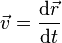$\vec{v} = {\mathrm{d}\vec{r} \over \mathrm{d}t}\,\!$.

In classical mechanics, velocities are directly additive and subtractive. For example, if one car traveling East at 60 km/h passes another car traveling East at 50 km/h, then from the perspective of the slower car, the faster car is traveling east at 60 − 50 = 10 km/h. Whereas, from the perspective of the faster car, the slower car is moving 10 km/h to the West. Velocities are directly additive as vector quantities; they must be dealt with using vector analysis.

Mathematically, if the velocity of the first object in the previous discussion is denoted by the vector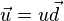$\vec{u} = u\vec{d}$ and the velocity of the second object by the vector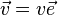$\vec{v} = v\vec{e}$ where$u$ is the speed of the first object,$v$ is the speed of the second object, and$\vec{d}$ and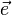$\vec{e}$ are unit vectors in the directions of motion of each particle respectively, then the velocity of the first object as seen by the second object is: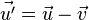$\vec{u'} = \vec{u} - \vec{v}\,\!$

Similarly:$\vec{v'}= \vec{v} - \vec{u}\,\!$

When both objects are moving in the same direction, this equation can be simplified to: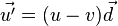$\vec{u'} = ( u - v ) \vec{d}\,\!$

Or, by ignoring direction, the difference can be given in terms of speed only: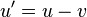$u' = u - v \,\!$

#### Acceleration

The acceleration, or rate of change of velocity, is the derivative of the velocity with respect to time (the second derivative of the position with respect to time) or$\vec{a} = {\mathrm{d}\vec{v} \over \mathrm{d}t}$.

Acceleration can arise from a change with time of the magnitude of the velocity or of the direction of the velocity or both. If only the magnitude,$v$, of the velocity decreases, this is sometimes referred to as deceleration, but generally any change in the velocity with time, including deceleration, is simply referred to as acceleration.

#### Frames of reference

While the position and velocity and acceleration of a particle can be referred to any observer in any state of motion, classical mechanics assumes the existence of a special family of reference frames in terms of which the mechanical laws of nature take a comparatively simple form. These special reference frames are called inertial frames. They are characterized by the absence of acceleration of the observer and the requirement that all forces entering the observer's physical laws originate in identifiable sources (charges, gravitational bodies, and so forth). A non-inertial reference frame is one accelerating with respect to an inertial one, and in such a non-inertial frame a particle is subject to acceleration by fictitious forces that enter the equations of motion solely as a result of its accelerated motion, and do not originate in identifiable sources. These fictitious forces are in addition to the real forces recognized in an inertial frame. A key concept of inertial frames is the method for identifying them. (See inertial frame of reference for a discussion.) For practical purposes, reference frames that are unaccelerated with respect to the distant stars are regarded as good approximations to inertial frames.

The following consequences can be derived about the perspective of an event in two inertial reference frames,$S$ and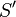$S'$, where$S'$ is traveling at a relative velocity of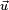$\scriptstyle{\vec{u}}$ to$S$.

•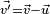$\scriptstyle{\vec{v'} = \vec{v} - \vec{u}}$ (the velocity$\scriptstyle{\vec{v'}}$ of a particle from the perspective of S' is slower by$\scriptstyle{\vec{u}}$ than its velocity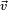$\scriptstyle{\vec{v}}$ from the perspective of S)
•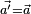$\scriptstyle{\vec{a'} = \vec{a}}$ (the acceleration of a particle remains the same regardless of reference frame)
•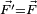$\scriptstyle{\vec{F'} = \vec{F}}$ (the force on a particle remains the same regardless of reference frame)
• the speed of light is not a constant in classical mechanics, nor does the special position given to the speed of light in relativistic mechanics have a counterpart in classical mechanics.
• the form of Maxwell's equations is not preserved across such inertial reference frames. However, in Einstein's theory of special relativity, the assumed constancy (invariance) of the vacuum speed of light alters the relationships between inertial reference frames so as to render Maxwell's equations invariant.

### Forces; Newton's Second Law

Newton was the first to mathematically express the relationship between force and momentum. Some physicists interpret Newton's second law of motion as a definition of force and mass, while others consider it to be a fundamental postulate, a law of nature. Either interpretation has the same mathematical consequences, historically known as "Newton's Second Law":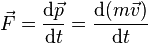$\vec{F} = {\mathrm{d}\vec{p} \over \mathrm{d}t} = {\mathrm{d}(m \vec{v}) \over \mathrm{d}t}$.

The quantity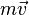$m\vec{v}$ is called the (canonical) momentum. The net force on a particle is, thus, equal to rate change of momentum of the particle with time. Since the definition of acceleration is$\vec{a} = \frac {\mathrm{d} \vec{v}} {\mathrm{d}t}$, when the mass of the object is fixed, for example, when the mass variation with velocity found in special relativity is negligible (an implicit approximation in Newtonian mechanics), Newton's law can be written in the simplified and more familiar form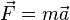$\vec{F} = m \vec{a}$.

So long as the force acting on a particle is known, Newton's second law is sufficient to describe the motion of a particle. Once independent relations for each force acting on a particle are available, they can be substituted into Newton's second law to obtain an ordinary differential equation, which is called the equation of motion.

As an example, assume that friction is the only force acting on the particle, and that it may be modeled as a function of the velocity of the particle, for example: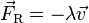$\vec{F}_{\rm R} = - \lambda \vec{v}$

with λ a positive constant.. Then the equation of motion is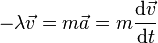$- \lambda \vec{v} = m \vec{a} = m {\mathrm{d}\vec{v} \over \mathrm{d}t}$.

This can be integrated to obtain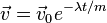$\vec{v} = \vec{v}_0 e^{- \lambda t / m}$

where$\vec{v}_0$ is the initial velocity. This means that the velocity of this particle decays exponentially to zero as time progresses. In this case, an equivalent viewpoint is that the kinetic energy of the particle is absorbed by friction (which converts it to heat energy in accordance with the conservation of energy), slowing it down. This expression can be further integrated to obtain the position$\vec{r}$ of the particle as a function of time.

Important forces include the gravitational force and the Lorentz force for electromagnetism. In addition, Newton's third law can sometimes be used to deduce the forces acting on a particle: if it is known that particle A exerts a force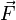$\vec{F}$ on another particle B, it follows that B must exert an equal and opposite reaction force, -$\vec{F}$, on A. The strong form of Newton's third law requires that$\vec{F}$ and -$\vec{F}$ act along the line connecting A and B, while the weak form does not. Illustrations of the weak form of Newton's third law are often found for magnetic forces.

### Energy

If a force$\vec{F}$ is applied to a particle that achieves a displacement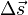$\Delta\vec{s}$, the work done by the force is defined as the scalar product of force and displacement vectors: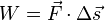$W = \vec{F} \cdot \Delta \vec{s}$.

If the mass of the particle is constant, and Wtotal is the total work done on the particle, obtained by summing the work done by each applied force, from Newton's second law:$W_{\rm total} = \Delta E_k \,\!$,

where Ek is called the kinetic energy. For a point particle, it is mathematically defined as the amount of work done to accelerate the particle from zero velocity to the given velocity v: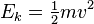$E_k = \begin{matrix} \frac{1}{2} \end{matrix} mv^2$.

For extended objects composed of many particles, the kinetic energy of the composite body is the sum of the kinetic energies of the particles.

A particular class of forces, known as conservative forces, can be expressed as the gradient of a scalar function, known as the potential energy and denoted Ep: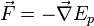$\vec{F} = - \vec{\nabla} E_p$.

If all the forces acting on a particle are conservative, and Ep is the total potential energy (which is defined as a work of involved forces to rearrange mutual positions of bodies), obtained by summing the potential energies corresponding to each force$\vec{F} \cdot \Delta \vec{s} = - \vec{\nabla} E_p \cdot \Delta \vec{s} = - \Delta E_p \Rightarrow - \Delta E_p = \Delta E_k \Rightarrow \Delta (E_k + E_p) = 0 \,\!$.

This result is known as conservation of energy and states that the total energy,$\sum E = E_k + E_p \,\!$

is constant in time. It is often useful, because many commonly encountered forces are conservative.

### Beyond Newton's Laws

Classical mechanics also includes descriptions of the complex motions of extended non-pointlike objects. The concepts of angular momentum rely on the same calculus used to describe one-dimensional motion.

There are two important alternative formulations of classical mechanics: Lagrangian mechanics and Hamiltonian mechanics. These, and other modern formulations, usually bypass the concept of "force," instead referring to other physical quantities, such as energy, for describing mechanical systems.

### Classical transformations

Consider two reference frames S and S' . For observers in each of the reference frames an event has space-time coordinates of (x,y,z,t) in frame S and (x' ,y' ,z' ,t' ) in frame S' . Assuming time is measured the same in all reference frames, and if we require x = x' when t = 0, then the relation between the space-time coordinates of the same event observed from the reference frames S' and S, which are moving at a relative velocity of u in the x direction is:

x' = x - ut
y' = y
z' = z
t' = t

This set of formulas defines a group transformation known as the Galilean transformation (informally, the Galilean transform). This group is a limiting case of the Poincaré group used in special relativity. The limiting case applies when the velocity u is very small compared to c, the speed of light.

For some problems, it is convenient to use rotating coordinates (reference frames). Thereby one can either keep a mapping to a convenient inertial frame, or introduce additionally a fictitious centrifugal force and Coriolis force.

## Limits of validity

Many branches of classical mechanics are simplifications or approximations of more accurate forms; two of the most accurate being general relativity and relativistic statistical mechanics. Geometric optics is an approximation to the quantum theory of light, and does not have a superior "classical" form.

### The Newtonian approximation to special relativity

Newtonian, or non-relativistic classical momentum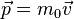$\vec{p} = m_0 \vec{v}$

is the result of the first order Taylor approximation of the relativistic expression:$\vec{p} = \frac{m_0 \vec{v}}{ \sqrt{1-v^2/c^2}} = m_0 \vec{v} \left(1+\frac{1}{2}\frac{v^2}{c^2} + ... \right)$, where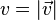$v=|\vec{v}|$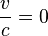$\frac{v}{c}=0$

so it is only valid when the velocity is much less than the speed of light. Quantitatively speaking, the approximation is good so long as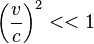$\left(\frac{v}{c}\right)^2 << 1$

For example, the relativistic cyclotron frequency of a cyclotron, gyrotron, or high voltage magnetron is given by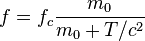$f=f_c\frac{m_0}{m_0+T/c^2}$, where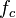$f_c$ is the classical frequency of an electron (or other charged particle) with kinetic energy$T$ and (rest) mass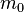$m_0$ circling in a magnetic field. The (rest) mass of an electron is 511 keV. So the frequency correction is 1 percent for a magnetic vacuum tube with a 5.11 kV. direct current accelerating voltage.

### The classical approximation to quantum mechanics

The ray approximation of classical mechanics breaks down when the de Broglie wavelength is not much smaller than other dimensions of the system. For non-relativistic particles, this wavelength is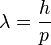$\lambda=\frac{h}{p}$

where h is Planck's constant and p is the momentum.

Again, this happens with electrons before it happens with heavier particles. For example, the electrons used by Clinton Davisson and Lester Germer in 1927, accelerated by 54 volts, had a wave length of 0.167 nm, which was long enough to exhibit a single diffraction side lobe when reflecting from the face of a nickel crystal with atomic spacing of 0.215 nm. With a larger vacuum chamber, it would seem relatively easy to increase the angular resolution from around a radian to a milliradian and see quantum diffraction from the periodic patterns of integrated circuit computer memory.

More practical examples of the failure of classical mechanics on an engineering scale are conduction by quantum tunneling in tunnel diodes and very narrow transistor gates in integrated circuits.

Classical mechanics is the same extreme high frequency approximation as geometric optics. It is more often accurate because it describes particles and bodies with rest mass. These have more momentum and therefore shorter De Broglie wavelengths than massless particles, such as light, with the same kinetic energies.

 General subfields within physics Atomic, molecular, and optical physics | Classical mechanics | Condensed matter physics | Continuum mechanics | Electromagnetism | General relativity | Particle physics | Quantum field theory | Quantum mechanics | Special relativity | Statistical mechanics | Thermodynamics

• History of classical mechanics
• Dynamical systems
• List of equations in classical mechanics
• Molecular dynamics
• Newton's laws of motion
• Special theory of relativity
• Dynamics
• Kinematics
• Statics
• Celestial Mechanics
• Continuum mechanics
• General Relativity
• Geometric Optics
• Hamiltonian Mechanics
• Lagrangian Mechanics
• Newtonian Mechanics
• Special Relativity
• Statistical Mechanics
• Thermodynamics